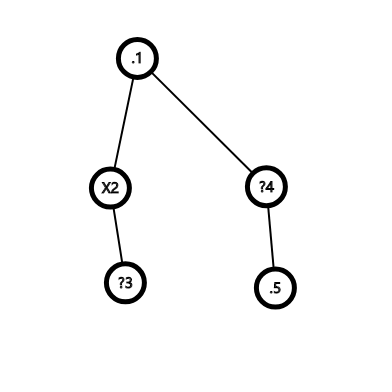# Roguelike Tree

## Statement

You are given a tree of N nodes. Each node could be 3 status: passable(.), impassable(X), unknown(?).

Now we randomly replace each "unknown" to "passable" or "impassable" then we need to find out the expected value of connected node pairs.

We call two nodes connected, if the path between them won't pass any impassable nodes.

## Examples

5
. X ? ? .
1 2
2 3
1 4
4 5

1.5

### Explain:If 3='.' 4='.' then pairs={1,4} {4,5} {1,5}
If 3='.' 4='X' then pairs=none
if 3='X' 4='.' then pairs={1,4} {4,5} {1,5}
if 3='X' 4='X' then pairs=none